# Deutsch-Jozsa算法

## 1.介绍

### 1.1 Deutsch-Jozsa问题

f(\{x_0,x_1,x_2,...\}) \rightarrow 0 \textrm{ or } 1 \textrm{ , where } x_n \textrm{ is } 0 \textrm{ or } 1

### 1.2 经典解决方法

P_\textrm{constant}(k) = 1 - \frac{1}{2^{k-1}} \qquad \textrm{for } 1 < k \leq 2^{n-1}

### 1.3量子解决方案

1.准备两个量子寄存器。第一个是初始化为 |0⟩n -量子比特寄存器，第二个是初始化为 |1⟩ 的单量子比特寄存器:

\vert \psi_0 \rangle = \vert0\rangle^{\otimes n} \vert 1\rangle

2.对每个量子比特应用哈达玛门:

\vert \psi_1 \rangle = \frac{1}{\sqrt{2^{n+1}}}\sum_{x=0}^{2^n-1} \vert x\rangle \left(|0\rangle - |1 \rangle \right)

3.应用量子oracle \vert x\rangle \vert y\rangle\vert x\rangle \vert y\ oplus f(x)\rangle:

\begin{aligned} \lvert \psi_2 \rangle & = \frac{1}{\sqrt{2^{n+1}}}\sum_{x=0}^{2^n-1} \vert x\rangle (\vert f(x)\rangle - \vert 1 \oplus f(x)\rangle) \\ & = \frac{1}{\sqrt{2^{n+1}}}\sum_{x=0}^{2^n-1}(-1)^{f(x)}|x\rangle ( |0\rangle - |1\rangle ) \end{aligned}

4.此时，第二个单量子比特寄存器可以被忽略。对第一个寄存器中的每个量子比特应用哈达玛门:

\begin{aligned} \lvert \psi_3 \rangle & = \frac{1}{2^n}\sum_{x=0}^{2^n-1}(-1)^{f(x)} \left[ \sum_{y=0}^{2^n-1}(-1)^{x \cdot y} \vert y \rangle \right] \\ & = \frac{1}{2^n}\sum_{y=0}^{2^n-1} \left[ \sum_{x=0}^{2^n-1}(-1)^{f(x)}(-1)^{x \cdot y} \right] \vert y \rangle \end{aligned}

5.测量第一个寄存器。请注意，计算 \vert 0 \rangle ^{\otimes n} = \lvert \frac{1}{2^n}\sum_{x=0}^{2^n-1}(-1)^{f(x)} \rvert^2 的概率，如果 f(x) 是常数，则计算为 1 ;如果 f(x) 是平衡的，则计算为 0

### 1.4 为什么会这样呢?

• 常数Oracle

H^{\otimes n}\begin{bmatrix} 1 \\ 0 \\ 0 \\ \vdots \\ 0 \end{bmatrix} = \tfrac{1}{\sqrt{2^n}}\begin{bmatrix} 1 \\ 1 \\ 1 \\ \vdots \\ 1 \end{bmatrix} \quad \xrightarrow{\text{after } U_f} \quad H^{\otimes n}\tfrac{1}{\sqrt{2^n}}\begin{bmatrix} 1 \\ 1 \\ 1 \\ \vdots \\ 1 \end{bmatrix} = \begin{bmatrix} 1 \\ 0 \\ 0 \\ \vdots \\ 0 \end{bmatrix}
• 平衡Oracle

U_f \tfrac{1}{\sqrt{2^n}}\begin{bmatrix} 1 \\ 1 \\ 1 \\ \vdots \\ 1 \end{bmatrix} = \tfrac{1}{\sqrt{2^n}}\begin{bmatrix} -1 \\ 1 \\ -1 \\ \vdots \\ 1 \end{bmatrix}

## 2. 举例

f(0,0)=0\\\\ f(0,1)=1\\\\ f(1,0)=1\\\\ f(1,1)=0

U_f \lvert x_1, x_0 \rangle = (-1)^{f(x_1, x_0)}\lvert x \rangle

\lvert \psi_0 \rangle = \lvert 0 0 \rangle_{01} \otimes \lvert 1 \rangle_{2}
1. 两个量子比特的第一个寄存器初始化为 |00⟩ ，第二个寄存器量子比特初始化为 |1⟩ (注意，我们使用下标0、1和2来索引量子比特。下标“01”表示包含量子比特0和1的寄存器的状态)
\lvert \psi_0 \rangle = \lvert 0 0 \rangle_{01} \otimes \lvert 1 \rangle_{2}
1. 对所有量子比特应用哈达玛
\lvert \psi_1 \rangle = \frac{1}{2} \left( \lvert 0 0 \rangle + \lvert 0 1 \rangle + \lvert 1 0 \rangle + \lvert 1 1 \rangle \right)_{01} \otimes \frac{1}{\sqrt{2}} \left( \lvert 0 \rangle - \lvert 1 \rangle \right)_{2}
1. oracle函数可以实现为 \text{Q}_f = CX_{02}CX_{12}
\begin{align*} \lvert \psi_2 \rangle = \frac{1}{2\sqrt{2}} \left[ \lvert 0 0 \rangle_{01} \otimes \left( \lvert 0 \oplus 0 \oplus 0 \rangle - \lvert 1 \oplus 0 \oplus 0 \rangle \right)_{2} \\ + \lvert 0 1 \rangle_{01} \otimes \left( \lvert 0 \oplus 0 \oplus 1 \rangle - \lvert 1 \oplus 0 \oplus 1 \rangle \right)_{2} \\ + \lvert 1 0 \rangle_{01} \otimes \left( \lvert 0 \oplus 1 \oplus 0 \rangle - \lvert 1 \oplus 1 \oplus 0 \rangle \right)_{2} \\ + \lvert 1 1 \rangle_{01} \otimes \left( \lvert 0 \oplus 1 \oplus 1 \rangle - \lvert 1 \oplus 1 \oplus 1 \rangle \right)_{2} \right] \end{align*}
1. 简化后，我们得到以下结果:
\begin{aligned} \lvert \psi_2 \rangle & = \frac{1}{2\sqrt{2}} \left[ \lvert 0 0 \rangle_{01} \otimes \left( \lvert 0 \rangle - \lvert 1 \rangle \right)_{2} - \lvert 0 1 \rangle_{01} \otimes \left( \lvert 0 \rangle - \lvert 1 \rangle \right)_{2} - \lvert 1 0 \rangle_{01} \otimes \left( \lvert 0 \rangle - \lvert 1 \rangle \right)_{2} + \lvert 1 1 \rangle_{01} \otimes \left( \lvert 0 \rangle - \lvert 1 \rangle \right)_{2} \right] \\ & = \frac{1}{2} \left( \lvert 0 0 \rangle - \lvert 0 1 \rangle - \lvert 1 0 \rangle + \lvert 1 1 \rangle \right)_{01} \otimes \frac{1}{\sqrt{2}} \left( \lvert 0 \rangle - \lvert 1 \rangle \right)_{2} \\ & = \frac{1}{\sqrt{2}} \left( \lvert 0 \rangle - \lvert 1 \rangle \right)_{0} \otimes \frac{1}{\sqrt{2}} \left( \lvert 0 \rangle - \lvert 1 \rangle \right)_{1} \otimes \frac{1}{\sqrt{2}} \left( \lvert 0 \rangle - \lvert 1 \rangle \right)_{2} \end{aligned}
1. 在第一个寄存器上应用哈达玛
\lvert \psi_3\rangle = \lvert 1 \rangle_{0} \otimes \lvert 1 \rangle_{1} \otimes \left( \lvert 0 \rangle - \lvert 1 \rangle \right)_{2}
1. 测量前两个量子比特将得到非零值 11 ，表明函数是平衡的。

from qiskit_textbook.widgets import dj_widget
dj_widget(size="small", case="balanced")


## 3.创造量子Oracles

1. 如果f(x) = 0，则将 I 门应用于寄存器2中的量子比特。

2. 如果f(x) = 1，则将 X 门应用于寄存器2中的量子比特。Input states that output 0 Input States that output 1
000 001
011 100
101 010
110 111Input states that output 0 Input states that output 1
001 000
010 011
100 101
111 110

## 4. Qiskit实现

# initialization
import numpy as np

# importing Qiskit
from qiskit import IBMQ, Aer
from qiskit.providers.ibmq import least_busy
from qiskit import QuantumCircuit, assemble, transpile

# import basic plot tools
from qiskit.visualization import plot_histogram


# set the length of the n-bit input string.
n = 3


### 4.1 常数Oracle

# set the length of the n-bit input string.
n = 3

const_oracle = QuantumCircuit(n+1)

output = np.random.randint(2)
if output == 1:
const_oracle.x(n)

const_oracle.draw()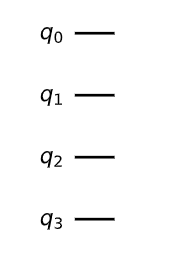### 4.2 平衡Oracle

balanced_oracle = QuantumCircuit(n+1)


b_str = "101"


balanced_oracle = QuantumCircuit(n+1)
b_str = "101"

# Place X-gates
for qubit in range(len(b_str)):
if b_str[qubit] == '1':
balanced_oracle.x(qubit)
balanced_oracle.draw()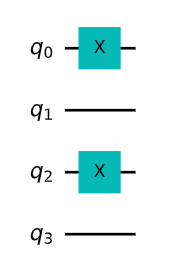balanced_oracle = QuantumCircuit(n+1)
b_str = "101"

# Place X-gates
for qubit in range(len(b_str)):
if b_str[qubit] == '1':
balanced_oracle.x(qubit)

# Use barrier as divider
balanced_oracle.barrier()

# Controlled-NOT gates
for qubit in range(n):
balanced_oracle.cx(qubit, n)

balanced_oracle.barrier()
balanced_oracle.draw()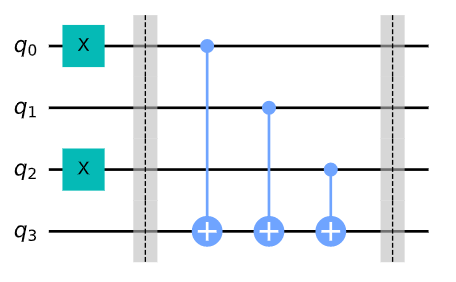balanced_oracle = QuantumCircuit(n+1)
b_str = "101"

# Place X-gates
for qubit in range(len(b_str)):
if b_str[qubit] == '1':
balanced_oracle.x(qubit)

# Use barrier as divider
balanced_oracle.barrier()

# Controlled-NOT gates
for qubit in range(n):
balanced_oracle.cx(qubit, n)

balanced_oracle.barrier()

# Place X-gates
for qubit in range(len(b_str)):
if b_str[qubit] == '1':
balanced_oracle.x(qubit)

# Show oracle
balanced_oracle.draw()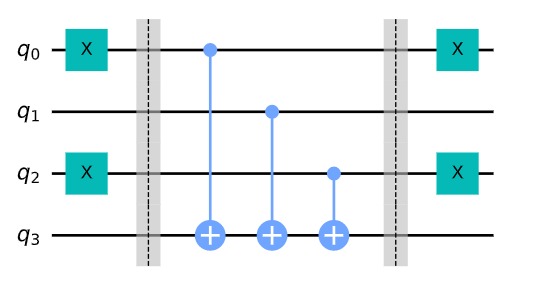## 4.3 完整算法

dj_circuit = QuantumCircuit(n+1, n)

# Apply H-gates
for qubit in range(n):
dj_circuit.h(qubit)

# Put qubit in state |->
dj_circuit.x(n)
dj_circuit.h(n)
dj_circuit.draw()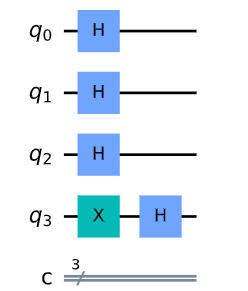dj_circuit = QuantumCircuit(n+1, n)

# Apply H-gates
for qubit in range(n):
dj_circuit.h(qubit)

# Put qubit in state |->
dj_circuit.x(n)
dj_circuit.h(n)

dj_circuit += balanced_oracle
dj_circuit.draw()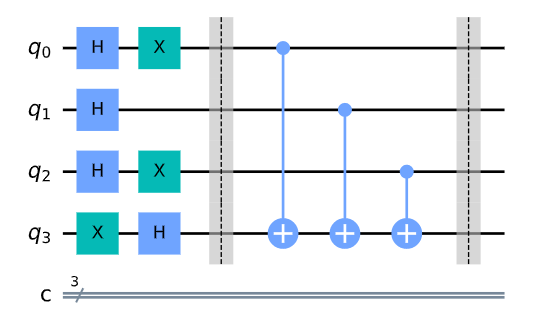dj_circuit = QuantumCircuit(n+1, n)

# Apply H-gates
for qubit in range(n):
dj_circuit.h(qubit)

# Put qubit in state |->
dj_circuit.x(n)
dj_circuit.h(n)

dj_circuit += balanced_oracle

# Repeat H-gates
for qubit in range(n):
dj_circuit.h(qubit)
dj_circuit.barrier()

# Measure
for i in range(n):
dj_circuit.measure(i, i)

# Display circuit
dj_circuit.draw()


# use local simulator
aer_sim = Aer.get_backend('aer_simulator')
qobj = assemble(dj_circuit, aer_sim)
results = aer_sim.run(qobj).result()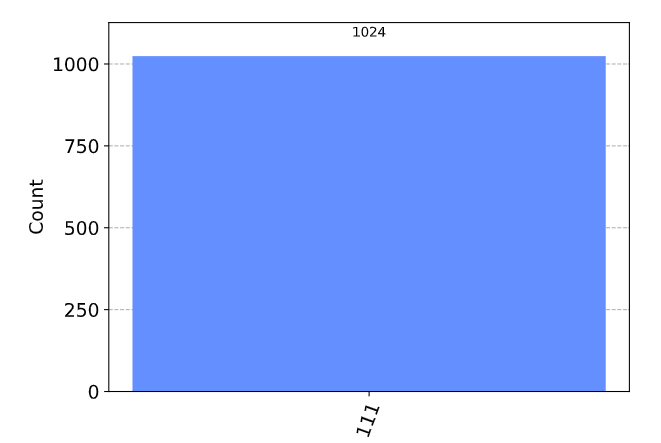## 4.4 广义电路

def dj_oracle(case, n):
# We need to make a QuantumCircuit object to return
# This circuit has n+1 qubits: the size of the input,
# plus one output qubit
oracle_qc = QuantumCircuit(n+1)

# First, let's deal with the case in which oracle is balanced
if case == "balanced":
# First generate a random number that tells us which CNOTs to
# wrap in X-gates:
b = np.random.randint(1,2**n)
# Next, format 'b' as a binary string of length 'n', padded with zeros:
b_str = format(b, '0'+str(n)+'b')
# Next, we place the first X-gates. Each digit in our binary string
# corresponds to a qubit, if the digit is 0, we do nothing, if it's 1
# we apply an X-gate to that qubit:
for qubit in range(len(b_str)):
if b_str[qubit] == '1':
oracle_qc.x(qubit)
# Do the controlled-NOT gates for each qubit, using the output qubit
# as the target:
for qubit in range(n):
oracle_qc.cx(qubit, n)
# Next, place the final X-gates
for qubit in range(len(b_str)):
if b_str[qubit] == '1':
oracle_qc.x(qubit)

# Case in which oracle is constant
if case == "constant":
# First decide what the fixed output of the oracle will be
# (either always 0 or always 1)
output = np.random.randint(2)
if output == 1:
oracle_qc.x(n)

oracle_gate = oracle_qc.to_gate()
oracle_gate.name = "Oracle" # To show when we display the circuit
return oracle_gate


def dj_algorithm(oracle, n):
dj_circuit = QuantumCircuit(n+1, n)
# Set up the output qubit:
dj_circuit.x(n)
dj_circuit.h(n)
# And set up the input register:
for qubit in range(n):
dj_circuit.h(qubit)
# Let's append the oracle gate to our circuit:
dj_circuit.append(oracle, range(n+1))
# Finally, perform the H-gates again and measure:
for qubit in range(n):
dj_circuit.h(qubit)

for i in range(n):
dj_circuit.measure(i, i)

return dj_circuit


n = 4
oracle_gate = dj_oracle('balanced', n)
dj_circuit = dj_algorithm(oracle_gate, n)
dj_circuit.draw()


transpiled_dj_circuit = transpile(dj_circuit, aer_sim)
qobj = assemble(transpiled_dj_circuit)
results = aer_sim.run(qobj).result()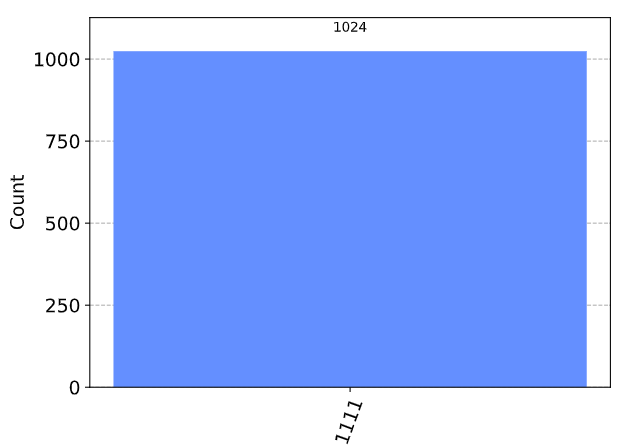## 5.真实设备实验

# Load our saved IBMQ accounts and get the least busy backend device with greater than or equal to (n+1) qubits
provider = IBMQ.get_provider(hub='ibm-q')
backend = least_busy(provider.backends(filters=lambda x: x.configuration().n_qubits >= (n+1) and
not x.configuration().simulator and x.status().operational==True))
print("least busy backend: ", backend)


# Run our circuit on the least busy backend. Monitor the execution of the job in the queue
from qiskit.tools.monitor import job_monitor

transpiled_dj_circuit = transpile(dj_circuit, backend, optimization_level=3)
job = backend.run(transpiled_dj_circuit)
job_monitor(job, interval=2)


# Get the results of the computation
results = job.result()



## 6.问题

1. 你能创造一个不同形式的平衡或常数的oracle吗?

2. 函数dj_problem_oracle(下面)以门的形式返回n = 4的Deutsch-Jozsa oracle。门接收5个量子比特作为输入，其中最后一个量子比特(q_4)是输出量子比特(如上面的oracle示例)。通过给dj_problem_oracle指定1到5之间的不同整数，可以得到不同的oracle。使用Deutsch-Jozsa算法来决定每个oracle是平衡的还是常数的(注意:强烈建议您使用aer_simulator而不是真正的设备来尝试这个例子)。

from qiskit_textbook.problems import dj_problem_oracle
oracle = dj_problem_oracle(1)


## 7.参考文献

1. David Deutsch and Richard Jozsa (1992). “Rapid solutions of problems by quantum computation”. Proceedings of the Royal Society of London A. 439: 553–558. doi:10.1098/rspa.1992.0167.

2. R. Cleve; A. Ekert; C. Macchiavello; M. Mosca (1998). “Quantum algorithms revisited”. Proceedings of the Royal Society of London A. 454: 339–354. doi:10.1098/rspa.1998.0164.